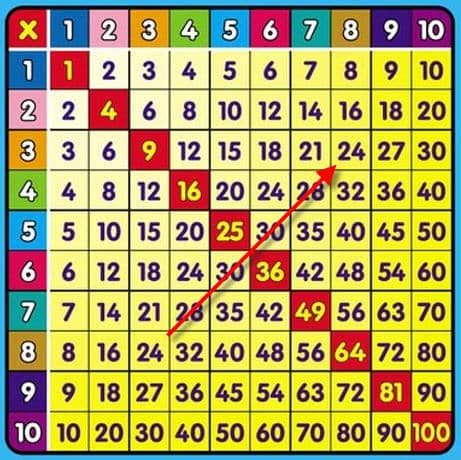# Tricks for Helping your Children Remember their Multiplication Math Facts

We know that committing the basic math facts to memory is a real chore for our kids. Surely there must be some logic to these sums. The answer is yes, there is. Here are some tricks for learning the multiplication math facts.

Let’s start by looking at the multiplication grid.Every multiplication has its twin. See, that 8x3, is the same as 3x8? Now, you’ll only have to remember half the table.

But there’s more.

Here are some more handy tips as your child starts learning each of the lines in the grid.

• To multiply by 2, you add the number to itself.

For example, 2 x 9 = 9 + 9

• To multiply by 5, the last digit of the sum always goes 5 or 0. It’s also always half of the 10x of the same number.

For example 10 x 4 = 40, 5 x 4 = 20, so 5 x 4 = half of 10 x 4.

• When you multiply 6 by an even number, they both end in the same digit.

Check it out: 6 x 2 = 12, 6 x 4 = 24, 6 x 6 = 36, 6 x 8 = 48.

• To multiply by 9, is 10x the number minus the number.

For example, 9 x 6 = 10 x 6 - 6 = 60 - 6 = 54.

• To multiply by 10, the last digit of the sum is always 0.
• To multiply by 11, all the way up to 9 x 11, just repeat the digit.

For example, 11 x 6 = 66.

• To multiply by 12, is the same as adding the multiplying by 10 to the multiplying by 2.

For example, 12 x 2 = 10 x 2 + 2 x 2 = 20 + 4 = 24.Become a Member

This content is available to members only.